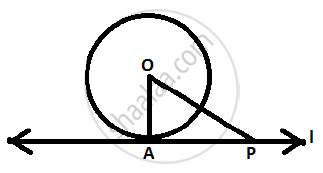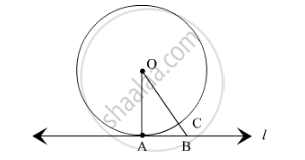# Prove that the Tangent at Any Point of a Circle is Perpendicular to the Radius Through The Point of Contact. - Mathematics

Prove that the tangent at any point of a circle is perpendicular to the radius through the point of contact.

#### Solution 1Given: Line is tangent to the (O, r) at point A.
To prove: bar(OA) _|_ l
Proof: Let P ∈ l  , P ≠ A.
If P is in the interior of (O,r), then the line will be a secant of the circle and not a tangent.
But l is a tangent of the circle, so P is not in the interior of the circle.

Also P≠A

P is the point in the exterior of the circle.

OP > OA ( bar(OA) is the radius of the circle )

Therefore each point P ∈ l except  A satisfies the inequality OP > OA.
Therefore OA is the shortest distance of line l from O.

bar(OA) _|_ l

#### Solution 2Given: A circle C (0, r) and a tangent l at point A

To prove: OA ⊥ l

Construction:

Take a point B, other than A, on the tangent l. Join OB. Suppose OB meets the circle in C.

Proof: We know that among all line segments joining the point O to a point on l, the perpendicular is shortest to l.

OA = OC  (Radius of the same circle)

Now,
OB = OC + BC

⇒ OB > OC

⇒ OB > OA

⇒ OA < OB

B is an arbitrary point on the tangent l. Thus, OA is shorter than any other line segment joining O to any point on l.

Here,

OA ⊥ l

Hence, the tangent at any point of a circle is perpendicular to the radius through the point of contact.

Is there an error in this question or solution?
2014-2015 (March) All India Set 2

Share